This video was brought to you by GeneralPAC.com, making power systems Intuitive, Open and Free for Everyone, Everywhere. Consider subscribing and supporting through patreon.com/GeneralPAC. This is a mechanism for you to support us financially so we can continue making high quality power system video tutorials. Our corporate sponsor for this topic is AllumiaX.com from Seattle, Washington. Contact them for industrial and commercial power system studies.

Introduction to the Delta Wye transformer connection part 2.

In part 1, we illustrated a very commonly connected delta wye transformer connection. In part 2, we'll look at the relationship between the line-to-line voltage and phase voltage for the Delta and Wye connection.

Check out the resources section under this video for helpful comments, suggestions, and clarifications.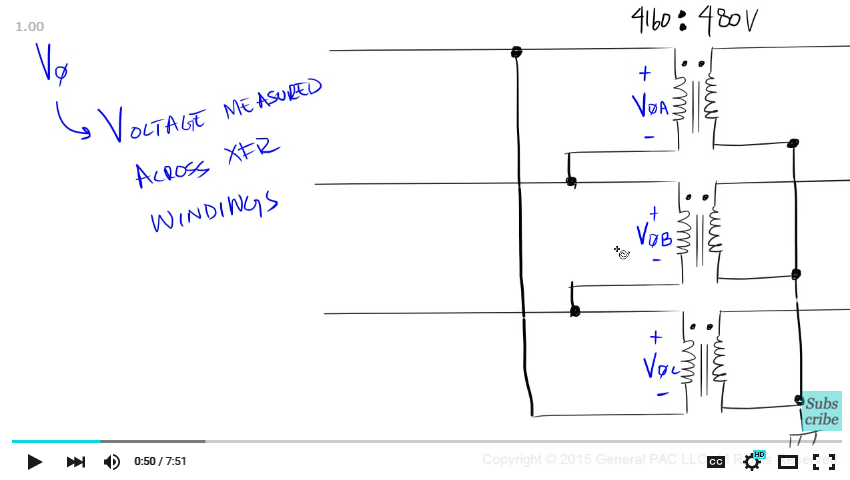We understand that phase voltage are voltage measured across transformer windings. Let's illustrate primary phase A voltage. Which is measured across the polarity side of winding A and the none polarity side of winding A. similarly, we'll illustrate primary phase B voltage and primary phase C voltage.

On the secondary side, we'll illustrate secondary phase a, secondary phase b voltage, and secondary phase c voltage. Line-to-line voltages however, are measured across two lines. The most common line-to-line voltage are measured across line a to line b. From line b to line c. and from line c to line a.

So on primary side where our transformer is connected in Delta. We should expect the line-to-line voltage across line A and line B to equal our phase A voltage.  Now it's very important to visually verify this relationship to get an intuitive understanding. Suppose we labeled point A and point B as the point of measurements.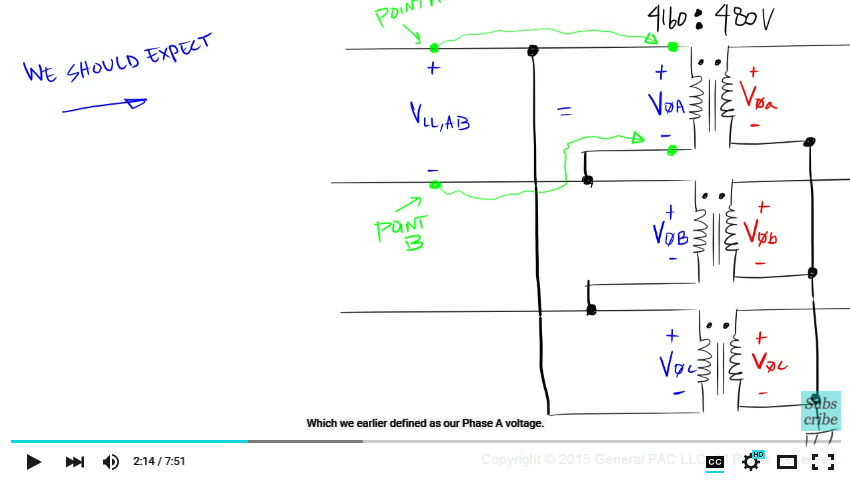Point A is the same point on the line as polarity side of winding A. And point B is the same point on the line as non-polarity side of winding A. So if we measure across point A and point B, we're actually measuring across the polarity side of winding A and the non-polarity side of winding A.  Which we earlier defined as our Phase A voltage.

Similarly, the line-to-line voltage across line B and line C is equal to phase B voltage. And the line-to-line voltage across line C and line A is equal to phase C voltage.

So for a Delta connected transformer, we should expect the voltage across two lines to equal the voltage across a single winding. Or in other words, the line-to-line voltage equals the phase voltage.

On the secondary side where our transformer is connected in wye. We'll have a different relationship between the line-to-line voltage and phase voltage. This relationship is discussed in great detail in a different module. So check out the resource section for more information.

The most common line-to-line measurements are across line a and line b. Across line b and line c. And lastly, across line a and line a.

The line-to-line voltage measurements across line a and line b is equal phase a voltage minus phase b voltage. For a Wye connected transformer, if we look at the magnitudes alone, we should expect the line-to-line voltage to equal the square root of 3 times the phase voltage. There is certainly going to be some phase shift involved between the two voltages. So if you look at the phase angle relationship, we should expect a 30 degrees phase shift between the line-to-line voltage and phase voltage. Again, this is covered in great detail in a different module.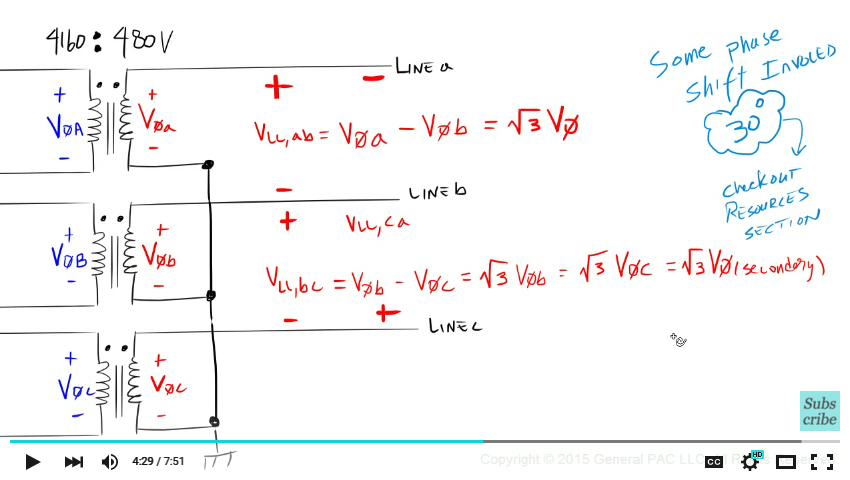So what's the line-to-line voltage between line b and line c? That's right, it's phase b voltage minus phase c voltage. Which is equal to the square root of 3 times the magnitude of phase b voltage. Which is also equal to the square root of 3 times the magnitude of phase c voltage. Or simply the square root of 3 times the magnitude of any phase voltage. And of course, we're assuming that we have a balanced set of voltages.

When I was first learning this, it helped me to visualize the relationship between the line-to-line voltage and phase voltage when looking at actual numbers. If we took a super duper volt meter and measured across line A and line B on the primary side of our transformer, we should expect 4160V. This is our line-to-line voltage we assumed in part 1.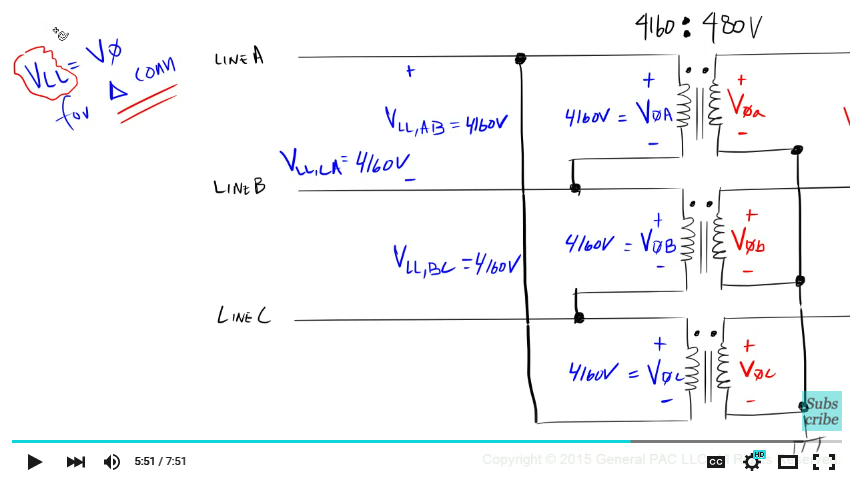Now since the primary side of our transformer is connected in delta, we should expect that the line-to-line voltage to equal our phase voltage. So we take our super duper volt meter and somehow get inside out transformer, and measure the difference in potential between the polarity side of winding A and none-polarity side of winding A, we should expect a voltage of 4160V. Similarly, the voltage across line B and line C is equal to 4160. And the line-to-line voltage across line C and line A should also equal 4160V. Both phase B and phase C should also equal 4160V.

So for a delta connected transformer, the line-to-line voltage is equal to the phase voltage. On the secondary side where our transformer is connected in Wye, if we measure the voltage across line A and line B we should expect 480V for the line-to-line voltage. Again, this is the voltage that we defined in Part 1. So what voltage should we expect if we measured across the polarity side of winding a and none-polarity side of winding a? Well we would have to derive that from the line-to-line voltage. Remember the relationship? For a wye connected transformer, the line-to-line voltage across line A and line B is equal to Phase a voltage minus Phase b voltage. Which is equal to the square root of three times any phase voltage. Since we already know that our line-to-line voltage is 480V, we simply solve for the phase voltage by dividing 480V by the square root of 3. Which gives us approximately 277V.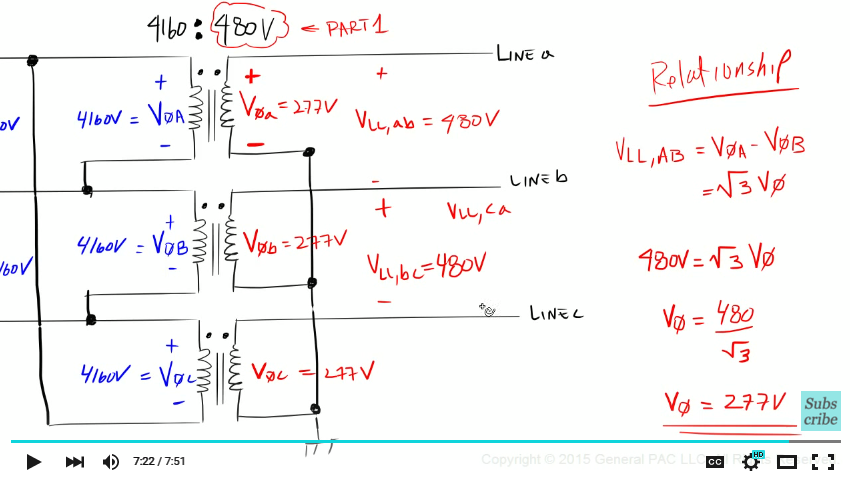So phase a voltage is equal to 277V. Similarly phase b voltage is equal to 277V.  And phase c voltage is also equal to 277V. And the line-to-line voltage across line b and line c, is equal to 480V. And the line-to-line voltage across line c and line a is equal to 480V.

In this part, we looked at the line-to-line voltage and phase voltage relationship between the delta wye transformer connections. In part 3, we'll look at the line current and phase current relationship.

This module was brought to you by GeneralPAC.com. Making Power System Protection, Automation, and Controls intuitive.

#### Greetings from the GeneralPAC Team!

We make high-quality Power Systems Video Tutorials on complex topics that are free and open to everyone!  Thank you so much for supporting us through Patreon so we can continue doing good and valuable work.

What is Patreon and why do we use it?

Patreon is a fantastic portal that allows our fans and community to make monthly contribution (like Netflix subscription) so we can continue creating high-quality power systems video tutorials. In return, you get access to incredible perks like voting on future topics, getting your questions answered, access to VIP Q/A webinars with the creators of GeneralPAC, and much more! We THANK YOU for supporting us

Why do we need your support?

An incredible amount of time and effort is needed to develop high-quality video tutorials. Each video (Part 1 for example) takes approximately 10 hours to complete which includes learning the concept ourselves, brainstorming creative ways to teach and explain the concepts, writing the script, audio recording, video recording, and editing. It's no wonder why Hundreds-of-Thousands of people have watched, liked, subscribed, and left positive comments on Youtube channel. Your support truly makes all the difference.